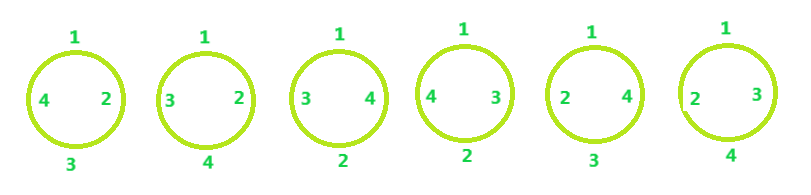# Permutations to arrange N persons around a circular table

• Difficulty Level : Medium
• Last Updated : 08 Jun, 2022

Given N, the number of persons. The task is to arrange N person around a circular table.
Examples

```Input: N = 4
Output: 6

Input: N = 5
Output: 24```

Approach: It is the concept of Circular permutation i.e. there is no specific starting point in the arrangement, any element can be considered as the start of the arrangement.
For N = 4, Arrangements will be:Below is the formula to find Circular permutations:

`Circular Permutations = (N - 1)!`

Below is the implementation of above idea:

## C++

 `// C++ code to demonstrate Circular Permutation` `#include ` `using` `namespace` `std;`   `// Function to find no. of permutations` `int` `Circular(``int` `n)` `{`   `    ``int` `Result = 1;`   `    ``while` `(n > 0) {` `        ``Result = Result * n;` `        ``n--;` `    ``}`   `    ``return` `Result;` `}`   `// Driver Code` `int` `main()` `{` `    ``int` `n = 4;`   `    ``cout << Circular(n - 1);` `}`

## Java

 `// Java code to demonstrate ` `// Circular Permutation ` `import` `java.io.*;`   `class` `GFG` `{` `// Function to find no. ` `// of permutations ` `static` `int` `Circular(``int` `n) ` `{ `   `    ``int` `Result = ``1``; `   `    ``while` `(n > ``0``) ` `    ``{ ` `        ``Result = Result * n; ` `        ``n--; ` `    ``} `   `    ``return` `Result; ` `} `   `// Driver Code` `public` `static` `void` `main(String[] args)` `{` `    ``int` `n = ``4``;` `    `  `    ``System.out.println(Circular(n - ``1``));` `}` `}`   `// This code is contributed ` `// by Naman_Garg`

## Python 3

 `# Python 3 Program to demonstrate Circular Permutation  `   `# Function to find no. of permutations` `def` `Circular(n) :` `    ``Result ``=` `1`   `    ``while` `n > ``0` `:` `        ``Result ``=` `Result ``*` `n` `        ``n ``-``=` `1`   `    ``return` `Result` `    `  `# Driver Code` `if` `__name__ ``=``=` `"__main__"` `:` `    ``n ``=` `4`   `    ``# function calling` `    ``print``(Circular(n``-``1``))`   `# This code is contributed by ANKITRAI1`

## C#

 `// C# code to demonstrate ` `// Circular Permutation ` `using` `System;` `                    `    `public` `class` `GFG {`   `// Function to find no. ` `// of permutations ` `static` `int` `Circular(``int` `n) ` `{ ` ` `  `    ``int` `Result = 1; ` ` `  `    ``while` `(n > 0) ` `    ``{ ` `        ``Result = Result * n; ` `        ``n--; ` `    ``} ` ` `  `    ``return` `Result; ` `} ` ` `  `// Driver Code` `public` `static` `void` `Main()` `{` `    ``int` `n = 4;` `     `  `    ``Console.Write(Circular(n - 1));` `}` `}` ` `  `/* This Java code is contributed by 29AjayKumar*/`

## PHP

 ` 0) ` `    ``{` `        ``\$Result` `= ``\$Result` `* ``\$n``;` `        ``\$n``--;` `    ``}`   `    ``return` `\$Result``;` `}`   `// Driver Code` `\$n` `= 4;`   `echo` `Circular(``\$n` `- 1);`   `// This code is contributed by mits` `?>`

## Javascript

 ``

Output:

`6`

Time Complexity: O(N) where N is the number of persons.
Auxiliary Space: O(1)

My Personal Notes arrow_drop_up
Recommended Articles
Page :